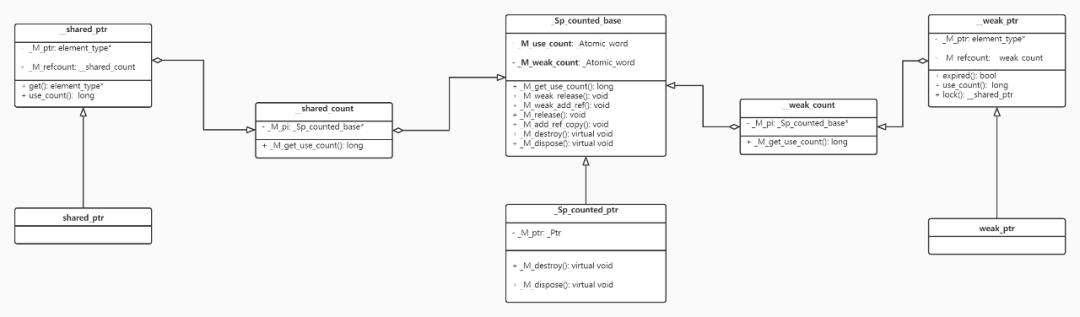# 源码分析 shared_ptr 实现• unique_ptr

• shared_ptr

• weak_ptr

#### shared_ptr的实现

﻿﻿

﻿

#### _M_use_count是如何加减的

_M_use_count表示托管对象的引用计数，即当shared_ptr拷贝时会增加，当shared_ptr析构时会减少，看代码：

template <typename _Yp>__shared_ptr(const __shared_ptr<_Yp, _Lp>& __r,                element_type* __p) noexcept    : _M_ptr(__p), _M_refcount(__r._M_refcount)  // never throws{}__shared_count(const __shared_count& __r) noexcept : _M_pi(__r._M_pi){    if (_M_pi != 0) _M_pi->_M_add_ref_copy();}template <>inline void _Sp_counted_base<_S_single>::_M_add_ref_copy(){    ++_M_use_count;}

﻿

template <typename _Yp>_Assignable<const shared_ptr<_Yp>&> operator=(    const shared_ptr<_Yp>& __r) noexcept{    this->__shared_ptr<_Tp>::operator=(__r);    return *this;}template <typename _Yp>_Assignable<_Yp> operator=(const __shared_ptr<_Yp, _Lp>& __r) noexcept{    _M_ptr = __r._M_ptr;    _M_refcount = __r._M_refcount;  // __shared_count::op= doesn't throw    return *this;}__shared_count& operator=(const __shared_count& __r) noexcept{    _Sp_counted_base<_Lp>* __tmp = __r._M_pi;    if (__tmp != _M_pi) {        if (__tmp != 0) __tmp->_M_add_ref_copy();        if (_M_pi != 0) _M_pi->_M_release();        _M_pi = __tmp;    }    return *this;}

﻿

_M_use_count是如何减为0的，可以猜想到shared_ptr析构时会调用__shared_count的析构函数，

﻿

~__shared_count() noexcept{    if (_M_pi != nullptr) _M_pi->_M_release();}template <>inline void _Sp_counted_base<_S_single>::_M_release() noexcept{    if (--_M_use_count == 0) {        _M_dispose();        if (--_M_weak_count == 0) _M_destroy();    }}virtual void _M_dispose() noexcept { delete _M_ptr; }

#### _M_weak_count是如何加减的

﻿

_Sp_counted_base() noexcept : _M_use_count(1), _M_weak_count(1) {}

﻿

__weak_count(const __weak_count& __r) noexcept : _M_pi(__r._M_pi){    if (_M_pi != nullptr) _M_pi->_M_weak_add_ref();}template <>inline void _Sp_counted_base<_S_single>::_M_weak_add_ref() noexcept{    ++_M_weak_count;}~__weak_count() noexcept{    if (_M_pi != nullptr) _M_pi->_M_weak_release();}template <>inline void _Sp_counted_base<_S_single>::_M_weak_release() noexcept{    if (--_M_weak_count == 0) _M_destroy();}virtual void _M_destroy() noexcept { delete this; }

﻿

#### weak_ptr的expired()和lock()做了什么

bool expired() const noexcept{    return _M_refcount._M_get_use_count() == 0;}

weak_ptr的expired()函数只是看了托管对象的引用计数是否为0，为0返回true

__shared_ptr<_Tp, _Lp> lock() const noexcept{    return __shared_ptr<element_type, _Lp>(*this, std::nothrow);}__shared_ptr(const __weak_ptr<_Tp, _Lp>& __r, std::nothrow_t)    : _M_refcount(__r._M_refcount, std::nothrow){    _M_ptr = _M_refcount._M_get_use_count() ? __r._M_ptr : nullptr;}

weak_ptr的lock()函数是打算返回一个shared_ptr对象来延长托管对象的生命周期，这里返回后需要判断返回值是否为nullptr。

shared_from_this()是如何实现的

class enable_shared_from_this{    shared_ptr<const _Tp> shared_from_this() const    {        return shared_ptr<const _Tp>(this->_M_weak_this);    }    mutable weak_ptr<_Tp> _M_weak_this;};

#### 总结

shared_ptr内部使用__shared_count中的_Sp_counted_base对象来控制托管指针，_Sp_counted_base内部有_M_use_count和_M_weak_count，_M_use_count表示托管指针的引用计数，_M_weak_count表示_Sp_counted_base的引用计数，_M_use_count为0时候释放托管指针指向的内存，_M_weak_count为0时释放_Sp_counted_base指向的内存，这里_Sp_counted_base的生命线一般不会短于shared_ptr的生命线。

﻿

﻿## 评论 (2 条评论)2020 年 05 月 07 日 18:38

2020 年 05 月 08 日 22:00